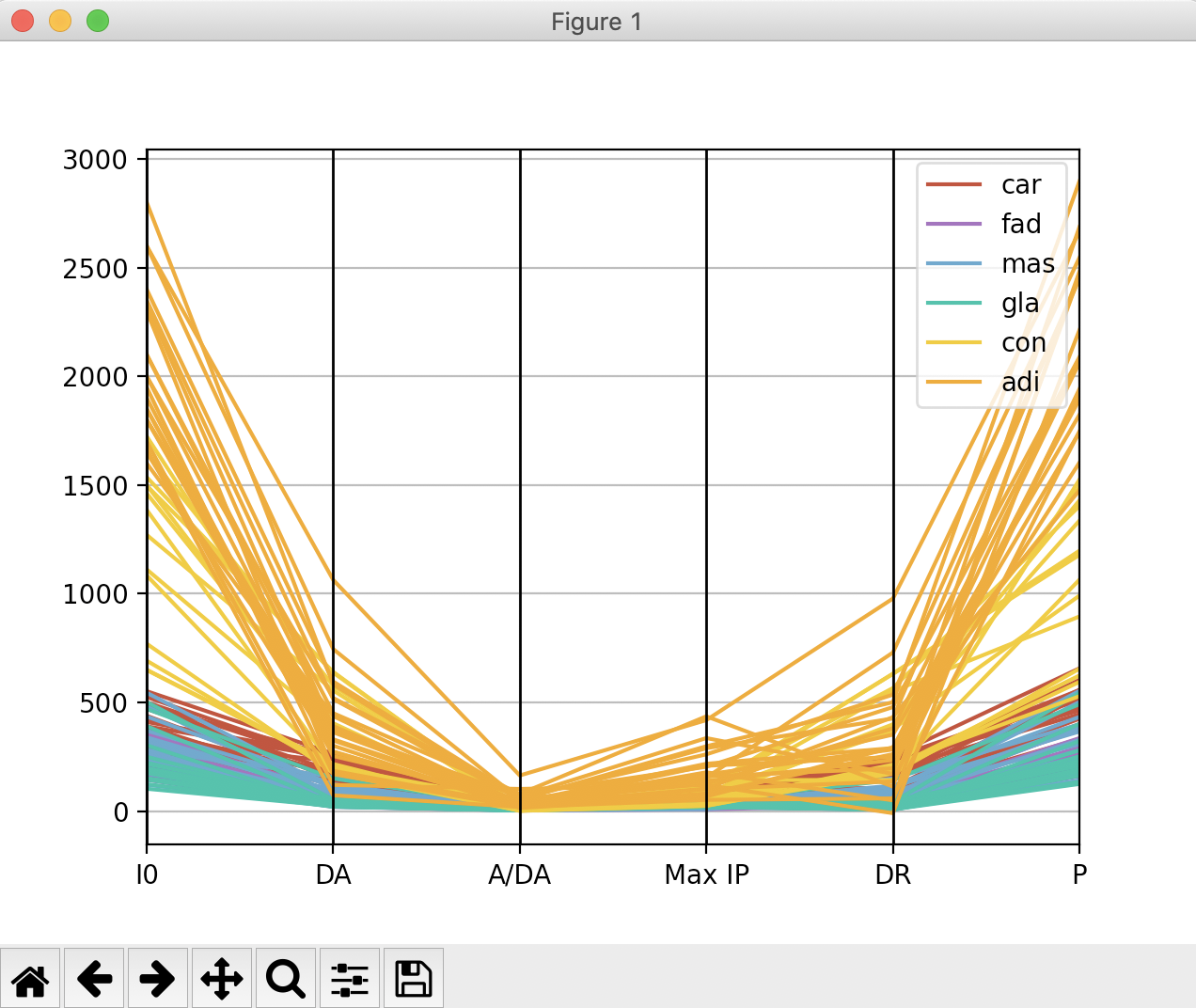# Parallel Coordinates Plotting Using Pandas

## Overview:

• Parallel coordinates is one of the oldest visualization techniques for understanding multivariate data.
• In a parallel coordinates plot, variables are represented through vertical parallel lines. These lines form the axes for the plot.
• Each data point of the multivariate data is marked in these vertical axes which result in a polyline.
• One data point corresponds to one polyline. In case of multiple data points with the same values, a poly line is drawn multiple times, one over another.
• The height of the vertical axes does not correspond to any order in the data.
• The parallel axis of the parallel coordinates plot can be drawn horizontally as well.
• The lines denoting the data point are typically not smooth as in case of Andrews Curves, which is another visualization technique that projects the multivariate data to a vector formed by the co-efficients of the finite Fourier series.

## Example:

 # Example Python program that creates # a parallel coordinates plot using pandas # plotting module # Data Courtesy: https://archive.ics.uci.edu/ml/datasets/Breast+Tissue import pandas as pds import matplotlib.pyplot as plt # Read data from a CSV file into a DataFrame df = pds.read_csv("BreastTissue.csv"); # Use the DataFrame instance and draw the parallel coordinates plot pds.plotting.parallel_coordinates(df, 'Class',                                   color=('#c0392b', '#9b59b6', '#5499c7',                                          '#1abc9c', '#f1c40f', '#f39c12')); plt.show(block=True);

## Output: